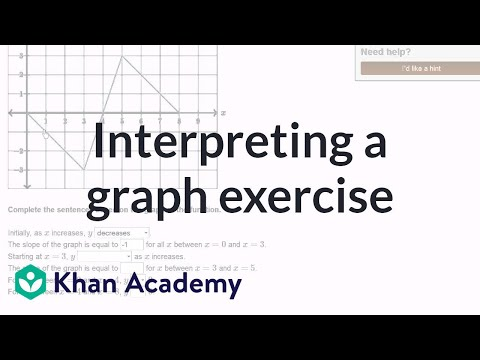# Graphs of piecewise linear functions

##By Khan Academy

Sal graphs a piecewise function given its formula. In this case, the function consists of three separate lines.# Graphs of piecewise linear functions

##By Khan Academy

Sal explains what a piecewise function is, and finds the formula of a piecewise function given its graph.# Interpreting graphs of linear and nonlinear functions

##By Khan Academy

Interpreting graphs of linear and nonlinear functions